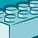# QlikView App Dev

Discussion Board for collaboration related to QlikView App Development.

Announcements
Now Live: Qlik Sense SaaS Simplified Authoring – Analytics Creation for Everyone: READ DETAILS
cancel
Showing results for
Did you mean:Creator

## How to show the percentage of the selected value over all values?

Dear Friends,

Suppose I have two columns (NAME and POINTS) like this:

 NAME POINTS John 4 Jack 3 Mary 6 Louis 2 Robert 1 Sam 7 John 5 Louis 3 Tom 9

with each name potentially appearing more than once.

I have built a small box to display the sum of points (merely SUM(POINTS)) and, when I select John in the above example, the box shows 9 (since 4 + 5 = 9).

Now, I wish to build another box, to display the percentage which this points represents over all the set of names. In the above example, since the column POINTS sums 40, if no one is selected, I want the box shows 100% (= 40/40) and, if I select John, I want the box shows 22,5% (= 9/40). Is it possible?

Labels (3)

• ### tables

1 Solution

Accepted SolutionsContributor III

Use this expression:

``sum(POINTS)/sum({<Name=>} POINTS)``

The denominator uses set analysis, {<Name=>}, to ignore the user's selection in the Name field, returning the total points.

This expression will ignore ALL selections, rather than just the user's selection in the Name field:

``sum(POINTS)/sum({1} POINTS)``

2 RepliesContributor III

Use this expression:

``sum(POINTS)/sum({<Name=>} POINTS)``

The denominator uses set analysis, {<Name=>}, to ignore the user's selection in the Name field, returning the total points.

This expression will ignore ALL selections, rather than just the user's selection in the Name field:

``sum(POINTS)/sum({1} POINTS)``